# PSEB 5th Class Maths Solutions Chapter 7 Geometry Ex 7.5

Punjab State Board PSEB 5th Class Maths Book Solutions Chapter 7 Geometry Ex 7.5 Textbook Exercise Questions and Answers.

## PSEB Solutions for Class 5 Maths Chapter 7 Geometry Ex 7.5

Question 1.
Give an examples of the following from the surroundings: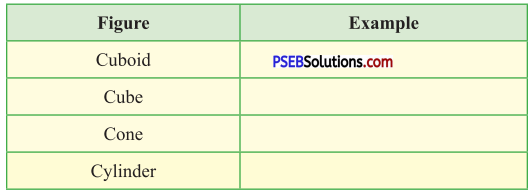Solution: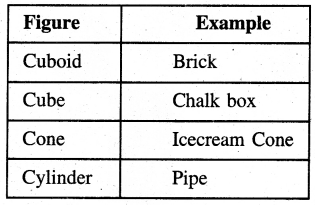Question 2.
Which of the following can form cubes ? Draw them on paper and find answers :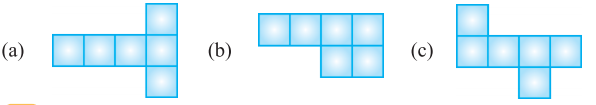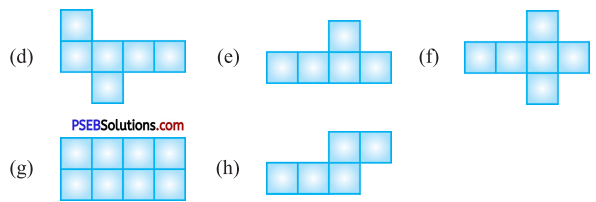Solution:
(a), (c), (d), (f).

3. Name the figures made from the following nets:

Question 1.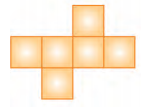Solution:
cube

Question 2.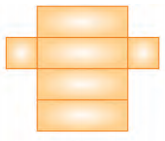Solution:
CuboidQuestion 3.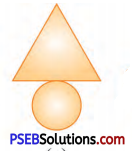Solution:
Cone

Question 4.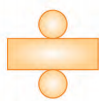Solution:
Cylinder

Question 4.
Match the following figures with their nets :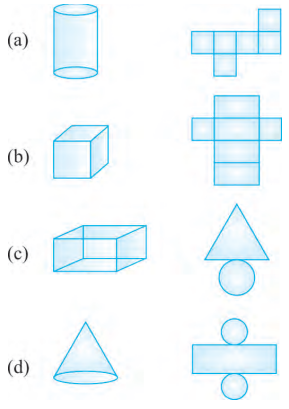Solution:
(a) – (iv)
(b) – (i)
(c) – (ii)
(d) – (iii).